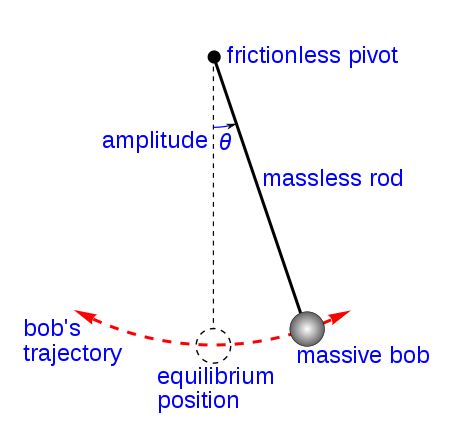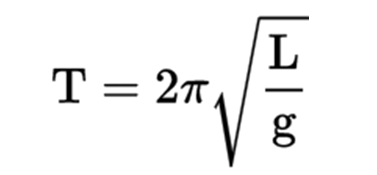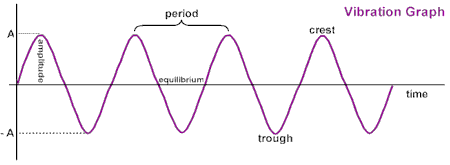# Pendulum Study Guide

INTRODUCTION:

You might have seen clocks like these used for antique or decorative purposes. We call it the pendulum clock. It has a dial that tells us the time, but a bob keeps swinging beneath it. Ever wondered why it moves like that? 🤔 Let’s study the pendulum and its motion in more detail.Source

## WHAT IS A PENDULUM?

A pendulum is a mass suspended from a pivot point that swings freely. When a pendulum is moved sideways from its resting equilibrium point, gravity acts as a restoring force, accelerating the pendulum towards its equilibrium position.Source

• The restoring force acting on the pendulum’s weight enables it to oscillate around the equilibrium position, swaying back and forth when released.
• The period is the time it takes to execute one complete cycle, including both left and right swings.
• The period is determined by the pendulum’s length and, to a lesser extent, by the amplitude or swing breadth.
• The equation for a pendulum’s period T is:• The motion made by a pendulum is an example of periodic motion. Curious what is periodic motion? Let’s know more.

## PERIODIC MOTION:

• Periodic motion is defined as a motion that replicates itself at regular time intervals.
• The motion by a pendulum can alternatively be classified as oscillatory.
• An oscillatory motion occurs when the body travels back and forth around a fixed point. As a result, an oscillatory motion can be periodic but is not required. A special case of oscillatory motion is simple harmonic motion.Source

## SIMPLE HARMONIC MOTION (SHM):

• SHM is a type of oscillation with a straight line connecting the two extreme locations.
• The entity’s path must be a straight line. A restoring force would be oriented towards the equilibrium (or mean) position.
• In simple harmonic motion, the mean position is a stable equilibrium.
• (examples of SHM motion as models).

# CONCLUSION

• Periodic motion is defined as a motion that replicates itself at regular time intervals.
• Simple harmonic motion is a subset of periodic motion where the restoring force on a traveling entity works in the object’s equilibrium point direction and is directly proportional to the object’s displacement.
• A pendulum is a mass suspended from a pivot point and may freely swing.

## FAQs:

1. How do pendulums work?

A pendulum is a weight that is suspended from a reference point and goes back and forth due to gravity. With no external assistance, the swing remains to swing back and forth until resistance slows it down and finally stops it.

2. What does it mean when a pendulum swings?

The restoring reaction force on the pendulum’s mass enables it to swing about the mean position, swinging back and forth when released. The period is the time it takes to complete one complete cycle, including both left and right swings.

3. What is the simple pendulum theory?

A basic pendulum comprises a mass m suspended on a string length L and a pivot point P. The pendulum is shifted to an initial angle and freed; it will swing back and forth regularly.

We hope you enjoyed studying this lesson and learned something cool about Pendulum! Join our Discord community to get any questions you may have answered and to engage with other students just like you! We promise, it makes studying much more fun! 😎

## SOURCES:

1. 10.3 Pendulum. https://www.ck12.org/book/peoples-physics-concepts/section/10.3/. Accessed 10 Feb 2022.
2. Pendulum. https://courses.lumenlearning.com/physics/chapter/16-4-the-simple-pendulum/. Accessed 10 Feb 2022.
]]>